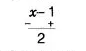# By actual division,`
Question:

By actual division, find the quotient and the remainder when the first

polynomial is divided by the second polynomial x4 + 1 and x-1.

Solution:

Using long division method,

$x-1) x^{4}+1\left(x^{3}+x^{2}+x+1\right.$

$\frac{x^{4}-x^{3}}{x^{3}+1}$

$\frac{x^{3}-x^{2}}{x^{2}+1}$

$\frac{x^{2}-x}{x+1}$Hence, Quotient $=x^{3}+x^{2}+x+1$ and Remainder $=2$